# Differential Calculus and the Geometry of Derivatives

Differential calculus is probably the greatest mathematical tool ever created for physics. It enabled Newton to develop his famous laws of dynamics in one of the greatest science book of all time, the Philosophiae Naturalis Principia Mathematica. Since then, differential calculus has had countless of other applications, like, for instance, in biodiversity, economics or optimization. It is hard to stress how essential it has become.

I usually write about grad school mathematics, but I have recently seen the following video about high school mathematics in the UK:

If you are interested by the question of what high school physics and mathematics should be like, you should check these two videos on Minute Physics and Sixty Symbols.

## Function

In textbooks, differential calculus is often illustrated by the calculation of speed given variations of position. This is also why it was first invented historically. But if you are rather an economist, you might have been introduced to calculus with the calculation of marginal cost, that is the variation of cost as a function of the amount of production. Let’s see something different in this article!

So what’s the example you’re going to use?

Let’s consider the following picture I took in the amazing Milford Sound, in New Zealand:Now, if you open this image with Windows preview application, iPhoto, GIMP or any image software, you’ll be able to modify the contrast of this picture (in image editing, you may find a Luminosity-Contrast option).

What’s the contrast?

Basically, as you increase the contrast, bright areas of the picture will become brighter, while dark areas will become darker. Now, computationally, the contrast is associated with a number. There are plenty of possible scales for the contrast, so I’ll use the one on iPhoto to illustrate what I’m saying. In iPhoto, the contrast is a number between -100 and +100. Let’s see what happens when I modify the contrast:iPhoto limits the contrast to the extremes -100 and 100, but there is no conceptual reason why we could not have even lower or higher contrasts. The lowest contrast would yield a totally grey image, while the highest contrast would produce a black and white image with no grey.

Now, as I’m manipulating this image, guess what my problem is…

I guess you’re wondering which contrast makes the most beautiful image?

Yes! But the problem with this question is that the concept of “beautiful” is not well defined. In order to do maths, I am going to introduce a measure of beauty. The first thing that would come to Mark Zuckerberg’s mind is probably the number of likes it would get once uploaded on social networks.

So what you want to study is how the contrast will affect the number of likes?

Exactly! There will be a number of likes corresponding to each contrast value. What we are defining here is a function which maps contrast values with number of likes.

Cool. But we don’t know what this function is unless we do the experiment…

Indeed. But since I’m too lazy to carry out the experiment, I’ll assume this function is given to us! This function is described by the following figure: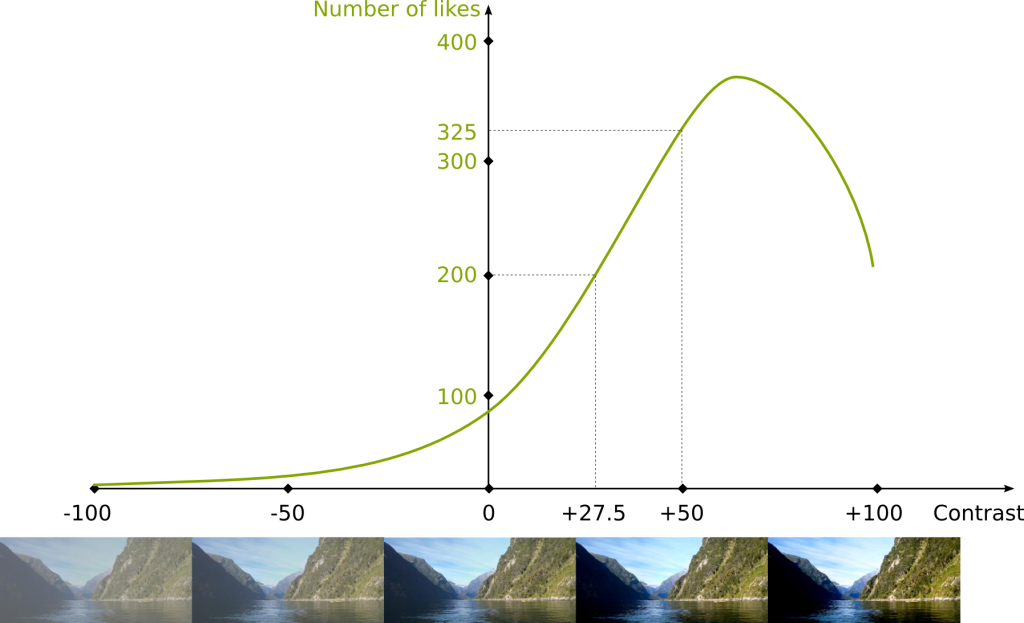Hundreds of likes? Really?

Obviously, it’s far from reality… But it’s the assumption we’re going to work with. In this figure you can see that any contrast value is mapped with a number of likes. For instance, if the contrast value is 50, then the number of likes is 325. Meanwhile, if the contrast is 27.5, then the number of likes is 200.

In practice, one way we can do to estimate this function is to do experiments for a few contrast values, and then, to find a function which is close to the results of the experiments. Such an operation is called an interpolation. If you can, please write about it.

## Derivative

Now that we have defined our problem, let’s get to the major concept of this article: differential calculus.

What is differential calculus?

It all starts with the following observation: Let’s zoom in around contrast value 27.5, as done in the following figure.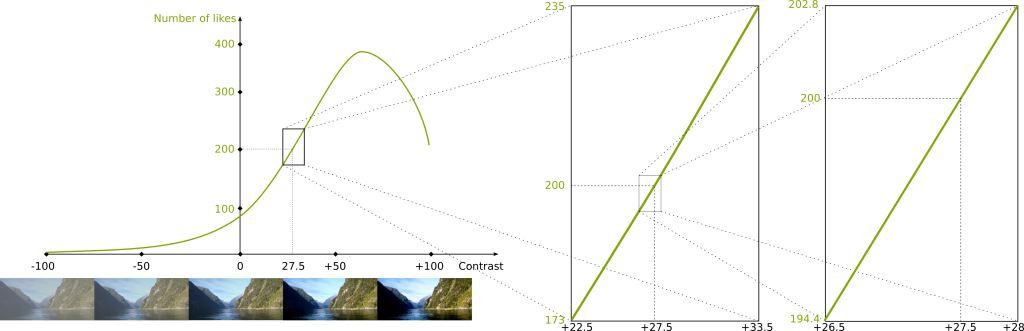Look at the most zoomed in picture on the right…

It looks like a line…

Exactly! This simple remark is the most important remark in calculus!

Really?

Yes! With more mathematical words, we say that the function can be locally approximated linearly. It’s the exact same reason why the Earth seems flat to us: It’s because our scale is much much smaller than the scale of the Earth. But as we know, the Earth isn’t flat, which has dramatic consequences, as explained by Scott.

Locally approximated linearly? This doesn’t sound very mathematical…

The actual mathematical definition of these terms is relatively complicated as it involves the fundamental concept of limits, which is a topological concept. You can read my article on topology to learn its most fundamental definition.

Here, let’s behave like 17th and 18th century mathematicians like Newton and Leibniz who used these concepts without actual good definition…

So zoomed-in curves look like lines… big deal!

It is a big deal!

Why?

Because lines are so much simpler than curves! While non-linear optimization is very difficult, linear programming can be done extremely quickly!

OK… Let’s study the local linear approximation around contrast value 27.5…

That’s the spirit! Now, one thing we already know about this line is that the number of likes equals 200 when the contrast value is 27.5. This defines a point through which the line must go. But this is not enough to describe the line. We need one more information. This information is the slope of the linear approximation.

What’s the slope?

The slope is more or less the direction of the line. The higher the slope, the more vertical the line. The slope can also be negative, in which case the line is going down. This is displayed by the following figure:So I guess that, in our case, the linear approximation corresponds to a positive slope…

Exactly!

But how can we find the exact value of the slope?

Let’s better define the slope! In our case, the slope is the variation of the number of likes for the linear approximation relatively to a variation of the contrast value. More generally and more precisely, the slope is the ratio of the variation of the value of linear approximation of the function by the variation of the argument of the function.

Those are long phrases… Can you show an example so I can understand?

Sure! Let’s look at the linear approximation we have obtained after having zoomed in.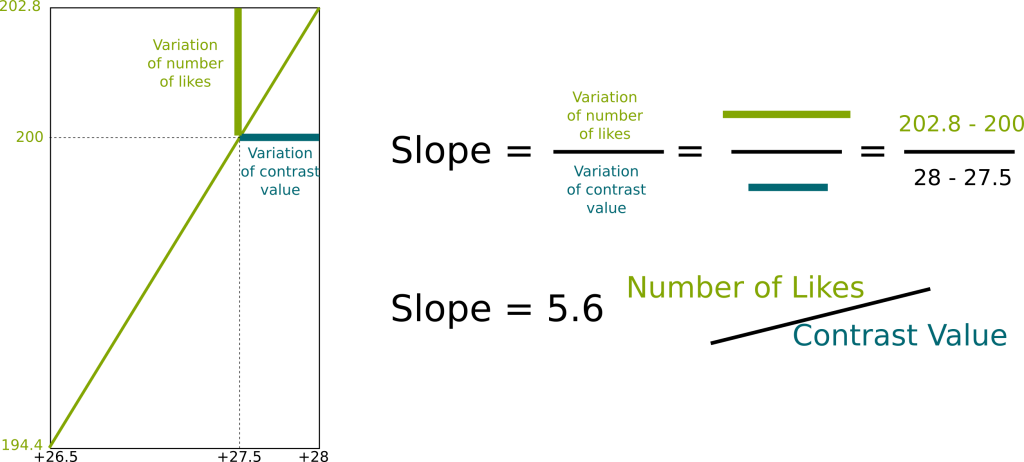Now, to compute the slope, we have to consider a variation of contrast value. For instance, let’s consider a variation of +0.5. This means that we are going to compare the reference contrast value 27.5 with the deviated contrast value 27.5+0.5 = 28. Now, to obtain the slope we need to estimate how this affects the number of likes. The reference number of likes is the number of likes corresponding to the reference contrast value 27.5, and as we have seen earlier, it is 200. Now the deviated number of likes is the one corresponding to the deviated contrast value 28. It is 202.8. This means that there has been a variation of number of likes equal to 202.8-200 = +2.8.

Overall, a variation of contrast value of +0.5 has induced a variation of number of likes of +2.8. The slope is the ratio of the effect of the variation by the variation. Thus, the slope of the linear approximation is 2.8/0.5 = 5.6.

So what does this number mean?

This is an excellent question! In fact, I strongly recommend you to question the meaning of any number you ever find. A bad interpretation of numbers is extremely misleading.

In our case, to obtain this number, we have divided a variation of number of likes by a variation of contrast value. So the unit of this number is a number of likes per contrast value. The slope is 5.6 likes per contrast value. It means that if we vary the contrast value by 1, then the number of likes will increase by 5.6.

But won’t this slope depend on the variation of contrast value we choose?

Not if you’re computing the slope of a line! Thus the slope of the linear approximation does not depend on the variation we choose.

But can’t we define slopes of functions by saying it’s the slope of the linear approximation?

Yes we can. The slope of the linear approximation of a function is called the derivative of the function. But it’s important to notice that the derivative depends on the contrast value where we compute it, because the linear approximation depends on this contrast value. The following figure shows linear approximations for different contrast values: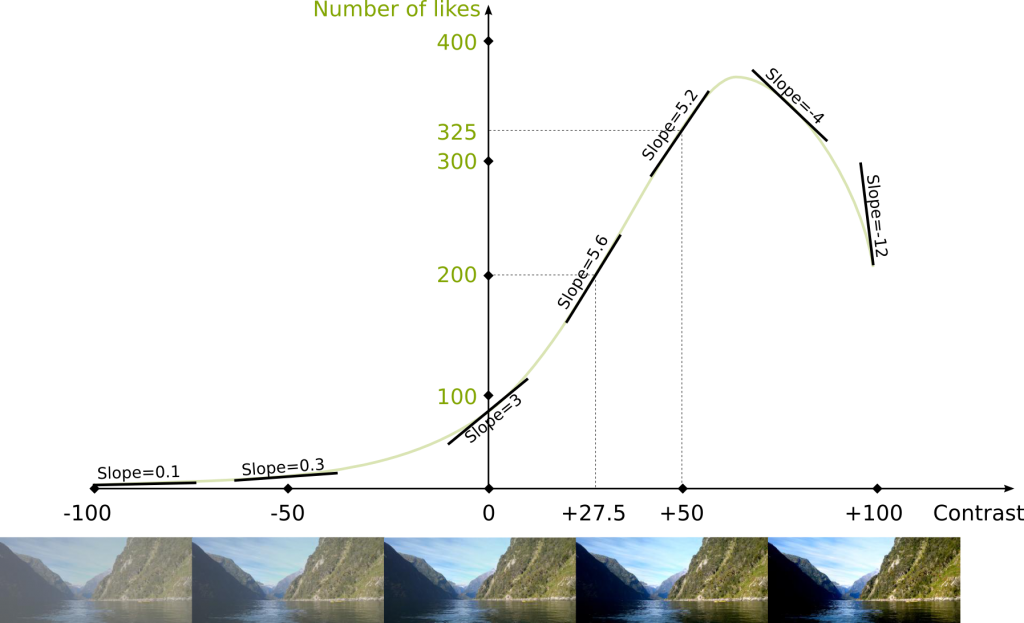Note that the linear approximation could be a vertical line, in which case there would be no real slope to describe it. We would say that the derivative isn’t defined for such point.

So there is a derivative for each contrast value…

This sounds like a function, doesn’t it? The derivative is actually the function that maps contrast values with slopes of the linear approximations. And we can draw this function, just like we drew our original function. In fact, one usual and nice way to do this is to draw the function on the same graph, but with a different vertical scale. Indeed, for instance, the unit must correspond to the unit of the derivative, as displayed in blue in the following figure: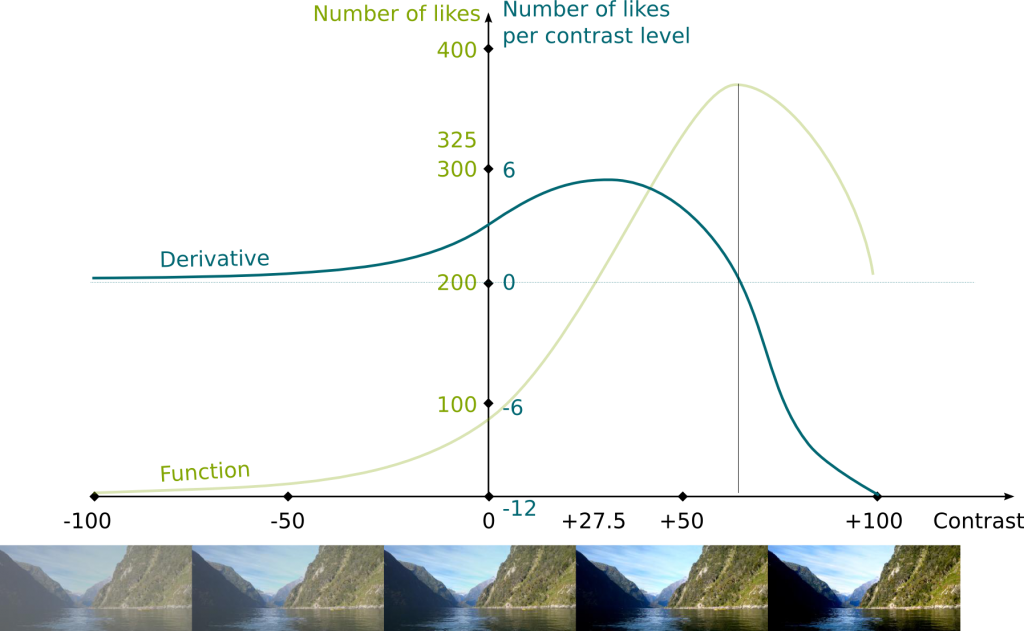How did you manage to obtain the derivative function?

Some of the value of the derivatives already appeared in the previous figure, and we just need to fill the gaps! But basically, the higher the slope of the linear approximation, the higher the derivative.

Now, if your function has an explicit form, like f(x)=x2 ln x, then there are formulas to obtain an explicit form of the derivative. But I won’t focus on these formulas in this article. If you can, please write an article about how to evaluate explicit forms of derivatives.

## Major Properties

As we have seen, the derivative is the slope of the linear approximation. And this slope tells us a big deal about the local monotonicity of the function.

What’s the monotonicity?

The monotonicity is whether the function is increasing, that is, whether increasing contrast will increase the number of likes. As you can see in the previous figures, if the slope of the linear approximation is positive, then this means that the function is increasing indeed.

This gives us the first major theorem about derivatives: the function is locally increasing if and only if its derivative is positive. Similarly, the function is locally decreasing if and only if its derivative is negative.

What about if the derivative is equal to zero?

Hehe! That’s more complicated. In fact, every case is possible as displayed in the following figures: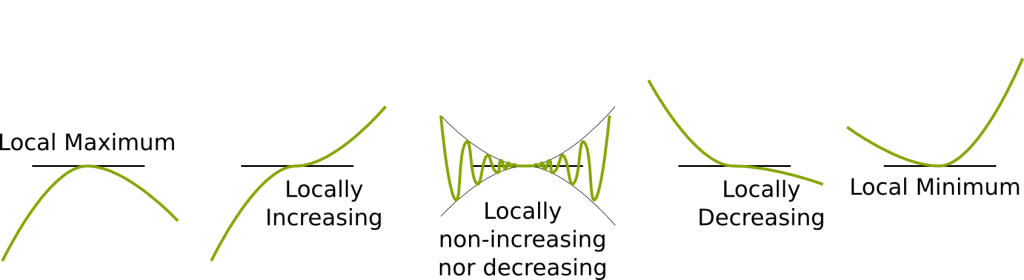Since people are often mistaken about nil derivatives, I’m going to write it in bold letters. If the derivative is nil, there’s not much we can say.

I’m confused… I learned that to find a maximum, we should search for nil derivatives… and you’re saying it’s useless?

The knowledge that the derivative is nil is useless. Now, an interesting consequence of the first major theorem we stated is the following. If a point is a maximum or a minimum, then its derivative can neither be positive nor negative: the derivative is therefore nil. This is the second major theorem you should know.

As a result, if you’re searching for the maximum of number of likes, you don’t need to test all the contrast values. You can restrict the search to the contrast values for which the derivative is nil. And most of the time, the number of values for which the derivative is nil is very small, so this leads you to a small number of tests.

So I should search for nil derivatives, just so that I only have a small number of values of the function to compare?

Exactly! Now, you can even better reduce the number of values to compare (possibly to one unique value), if you study the convexity of your function as well. But I’m not going to get into this right now. But if you can, you should write an article about convexity, which is a crucial concept in optimization.

We are now going to get into more complicated concepts of calculus. But don’t lose hope, I’m sure you can understand the following, and be more knowledgeable in calculus than Isaac Newton ever was!

Now, the second major theorem we mentioned has a consequence, called Rolle’s theorem. This is a major theorem in calculus.

What is it?

Before stating Rolle’s theorem, I want to stress something. The derivative of a function is only defined if there actually exists a linear approximation. This is not always the case. If the derivative is always defined, the function is called differentiable. That’s how nice classical functions are. But, for instance, the following function is not differentiable in 0, as there is no linear approximation at this point: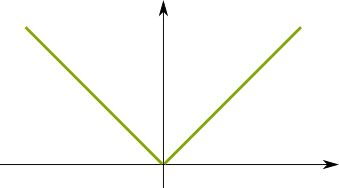Now, Rolle’s theorem says that if a function is differentiable between two extremity points, and if the values of the function at these two extremities are the same, then there exists a point between the two extremities which has a nil derivative.

Why?

One of these three cases is necessarily true (the first two may be both true).In any case, there is a maximum or a minimum between the extremities. According to the second major theorem, the derivative of this point is zero. This proof was given in 1691 by Michel Rolle.

Cool! Do I know more than Newton?

Well, not yet. Newton was still alive in 1691… Now, the fourth and last theorem I want to present in this article will make you more knowledgeable than the English genius! It’s the mean value theorem, proven by Augustin Louis Cauchy in the 19th century, and proudly written on this bridge in Beijing:What’s the mean value theorem?

Let’s consider two extremities once again, but without the assumption that they have the same values of the function. Now, we can consider the line which maps the function at the two extremities. This line is sort of a (very bad) approximation of the function between the two extremities, but its slope does describe how the function varies overall between the two extremities. Let’s call this slope, the overall slope of the function between the two extremities.

The mean value theorem says that, if the function is differentiable between the two extremities, then there exists a derivative between the extremities which is equal to the overall slope.

What???

This is illustrated in the following figure: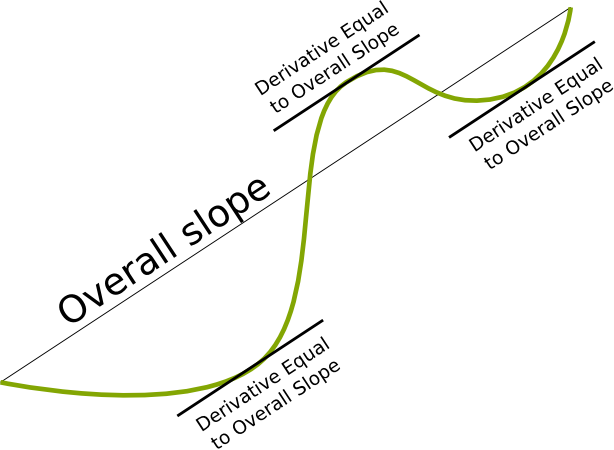I get it… But how do you prove it?

Just rotate the image so that the two extremities have the same value of the function! Let’s see what this yields: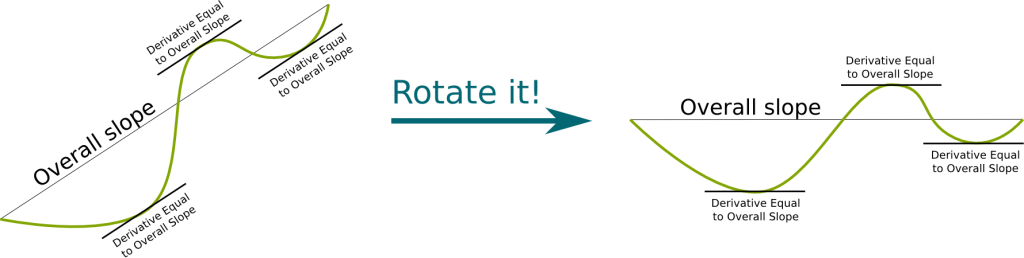This looks like Rolle’s theorem!

Indeed! We can apply Rolle’s theorem, which says that there is now a point between the extremities with a nil derivative. If we rotate back the figure, since we rotate all linear approximations with the same angles, then we see that the derivative of this point is actually equal to the overall slope.

This isn’t the actual proof, is it?

The actual proof consists in writing mathematically what we have done visually. This can be done by noticing that adding a linear term to a function is almost equivalent to rotating the figure. More precisely, it corresponds to a vertical contraction of the figure (I talk about contraction according to an axis in my article on symmetries). Based on this remark, I count on you to write the proof yourself!

In Rolle’s and the mean value theorem, for simplicity, I haven’t been rigorous regarding what I meant by “between”. More precisely, I haven’t explicitly said if I considered that the extremities were between the extremities… But I wanted to present the global idea. For a more rigorous understanding of these theorems, go through wikipedia’s articles!

## Let’s Conclude

I have introduced the derivative as the slope of the linear approximation, while you have probably learned about the limits of the difference quotients. But the concept of limits is very complicated (I have seen professors of mathematics actually make fundamental mistakes about this concept!), and I don’t want to introduce it without really explaining it. What I mean is that limits have to be treated with caution. Also, although in our simple cases, it doesn’t make much of a difference, in higher dimensions, understanding the derivative through the linear approximation is much more relevant.

The History of calculus is quite amusing, as it turned into a matter of national pride:

Th ideas of both scholars Newton and Leibniz were fantastic, but it’s interesting to notice that they were also not rigorous. In fact, it wasn’t until Cauchy in the early 1800s that the concept of derivative finally had a good definition! I think this must teach us humility. If even Isaac Newton couldn’t get his reasoning totally right, how can we claim that our reasonings are perfectly straight, unless based on extremely solid deductive proofs (which only exist in mathematics that only few understand)?

In fact, most of the time, we subconsciously consider something understood when it is not. One of the most striking example that comes to my mind regards the concept of acceleration. The derivative of the position is speed, and it is something we are relatively familiar with. But acceleration corresponds to the variation of speed. It’s therefore very different from speed! And we often make mistakes regarding it. For instance, keep in mind that the Moon is always accelerating towards the Earth, but never moving towards the Earth. I hope this can avoid your misconceptions. I really like the following video by Derek Muller on Veritasium about misconceptions:

## More on Science4All

The Magic of AnalysisBy Lê Nguyên Hoang | Updated:2016-02 | Views: 1884
This article retraces the endless pursuit of the infinite that is at the basis of mathematical analysis. From the first approximations of pi to the shape of our limitless universe, from the essential usefulness of differential equations to the troubles with infinite sums, we present the great ideas of mathematical geniuses all along History.

Topology: from the Basics to ConnectednessBy Lê Nguyên Hoang | Updated:2016-02 | Views: 7879
Topology was my favorite course in pure maths. I love it because it's a powerful abstract theory to describe intuitive and visual ideas about space. This article gives you an introduction to this amazing field. We'll introduce graph topology, metric spaces, continuity and connectedness.

Spacetime of General RelativityBy Lê Nguyên Hoang | Updated:2016-01 | Views: 13694
Most popular science explanations of the theory of general relativity are very nice-looking. But they are also deeply misleading. This article presents you a more accurate picture of the spacetime envisioned by Albert Einstein.

The Most Beautiful Equation of Math: Euler's IdentityBy Lê Nguyên Hoang | Updated:2016-01 | Views: 89747
In 1988, Euler's identity was elected most beautiful theorem of mathematics. It has been widely taught worldwide. But have you ever stopped to really sense the meaning of this incredible formula? This article does.